# Group Target Xbar-R Charts

Group target Xbar-R charts provide information about changes in process averages and the range of averages across multiple measurement subgroups of similar characteristics that have a common process. Review the following example—an excerpt from Innovative Control Charting1—to get a sense of how a group target Xbar-R chart works.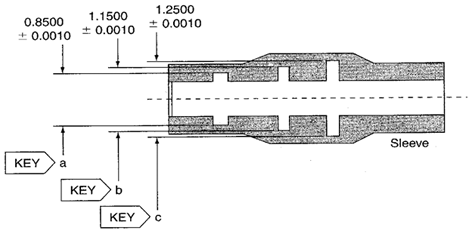Figure 1. Three sleeve-inside-diameter key characteristics.

## Case Description

This sleeve contains three inside diameter key characteristics. They are all machined on the same lathe but with different tools. Each inside diameter is a different size. The customer requires stability of the lathe process as well as capability information from each inside diameter.

## Sampling Strategy

Visibility is required of both process and part variability. Because the same type of characteristic (sleeve diameters) with different targets is being measured at multiple locations on the same part, a group target Xbar-R chart is selected. This chart will highlight both variation in the lathe and variation in each of the three sleeves.

The cycle time required to manufacture a sleeve is three minutes. Cutting tools are replaced about every two hours. The subgroups represent measurements taken every hour from three consecutive sleeves.

## Data Collection Sheet

Table 1. Group target Xbar-R chart data for three sleeve characteristics. MAX and MIN plot points are shown in bold.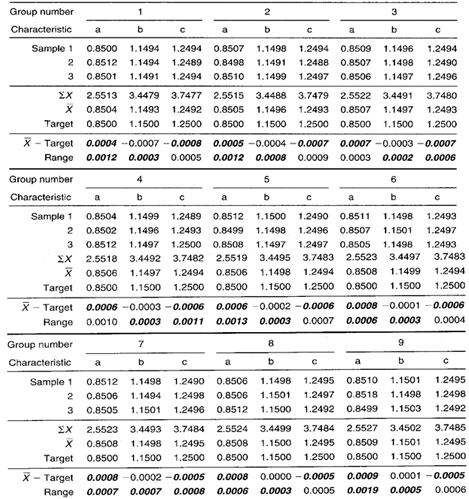## Group Target Xbar-R Chart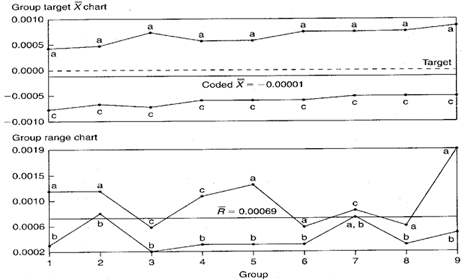Figure 2. Group target Xbar-R chart representing three different sized inside-sleeve diameters.

## Chart Interpretation

Group target range chart: Either characteristic a or c shows up in the MAX position in every group. This suggests that these two locations have the largest standard deviation values. Location b appears in the MIN position in every group. This means that, of the three diameters being evaluated, location b has the least variability.
Note: The centerline on the group range chart is the average of all the ranges in the data collection sheet.

Group target Xbar chart: Diameter a dominates the MAX position. It consistently deviates from its target (to the high side) more than the other diameters. Location c dominates the MIN position. It consistently deviates from its target (to the low side) more than the other diameters. Diameter b falls in between. It deviates from its target value less than diameters a or c. This is characteristic of taper in the diameters. Also, notice that the MAX and MIN lines are somewhat parallel and seem to gradually trend upwards.
Note: The centerline on the group target Xbar chart is the average of all the coded Xbar plot points in the data collection sheet.

## Recommendations

• Operators should find out why the diameters on the ends (a and c) have larger standard deviations. One might evaluate the cutting tools, the way the sleeve is held when machined, part loading techniques, wall thicknesses at the different locations, coolant flow, or measurement problems.
• People working in the process should try to eliminate the taper among the diameters. Change the process so that the a and c diameters fall closer to their targets.
• The upward trend on the Xbar chart appears to be a predictable tool wear condition. One may consider performing a regression analysis to estimate when the cutting tools should be replaced.

## Estimating the Process Average

If all of the key characteristics on the group target Xbar chart appeared to be behaving randomly, a single estimate of the process average could be used to estimate the process average for all locations. However, in this case, the group target Xbar chart does not exhibit random behavior.

Given this nonrandom behavior on the group target Xbar chart, estimates of the process average should be calculated separately for each characteristic on the group target chart. This is illustrated in Calculation 1 using data from diameter a.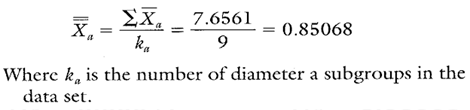Calculation 1. Estimate of the process average for diameter a.

## Estimating Sigma

Estimates of sigma arc also calculated separately for each characteristic on the group chart. Continuing with diameter a, see Calculations 2 and 3.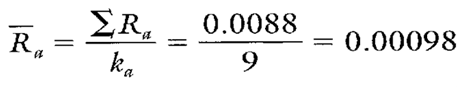Calculation 2. Calculation of R for use in estimating the process standard deviation for diameter a.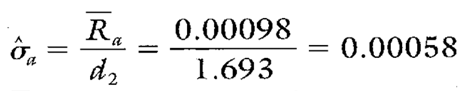Calculation 3. The estimate of the process standard deviation for diameter a.
Note: To ensure reliable estimates, k needs to be at least 20. In this example, k is only nine. Therefore, the estimates here and in Table 2 are for illustration purposes only.

## Calculating Process Capability and Performance Ratios

Calculations 4, 5, and 6 show the process capability and performance calculations for diameter a.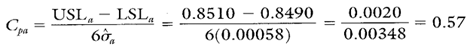Calculation 4. Cp calculation for diameter a.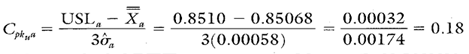Calculation 5. Cpk upper calculation for diameter a.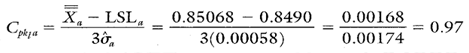Calculation 6. Cpk lower calculation for diameter a.

## Group Target Xbar-R Chart Advantages

• Simultaneously illustrates the variation of multiple product or process characteristics.
• Similar characteristics with different averages can be analyzed on the same chart.
• Separates variation due to changes in the average from variation due to changes in the standard deviation.
• Multiple characteristics can be tracked on one chart.

## Group Target Xbar-R Chart Disadvantages

• No visibility of the characteristics that fall between the MAX and MIN plot points.
• The use of negative numbers can be confusing.
• Cannot detect certain nonrandom conditions because the group charts described here have no control limits.

• The remaining process statistics and process capability and performance ratios for diameters b and c are shown in Table 2.
• Diameter a is not capable. Its average is greater than its target by almost 0.0007".
• Diameter b is capable although its average is more than 0.0002" lower than its target.
• Diameter c is not capable and its average is more than 0.0006" lower than its target.

Table 2. Additional statistics and process capability and performance values for diameters b and c.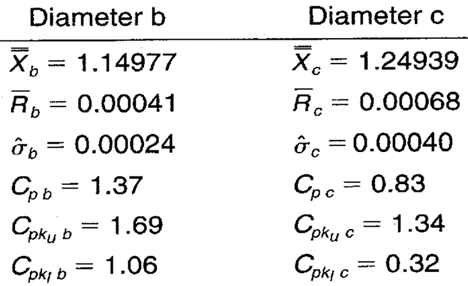When you use SPC software from InfinityQS, consuming the information provided by group target Xbar-R charts becomes faster and easier than ever. See how this type of analysis is surfaced in InfinityQS solutions.

FOOTNOTE:
1 Wise, Stephen A. and Douglas C. Fair. Innovative Control Charting: Practical SPC Solutions for Today’s Manufacturing Environment. Milwaukee, WI: ASQ Quality Press.

### Take the first step from quality to excellence

Take the Next Steps